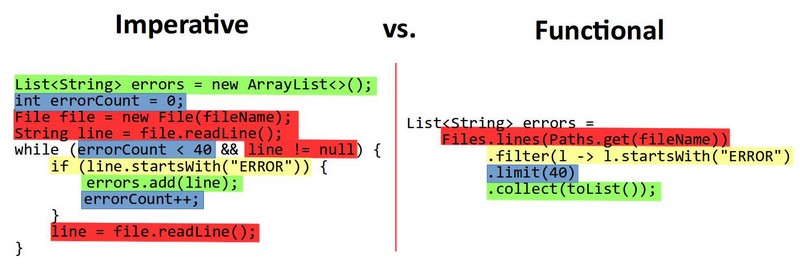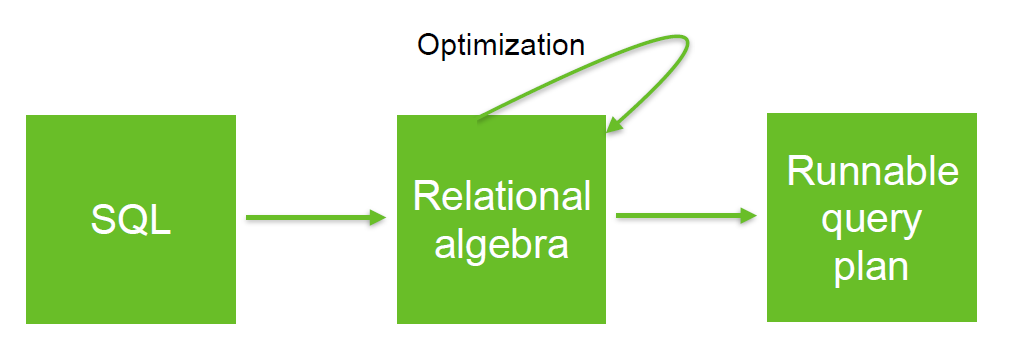# Functional Programming - Algebraic Data Type

An algebraic data type (wiki/Algebraic_data_type) is a data type that is the input and the output of its own operations.

## Benefits

• An algebraic structure can be composed before being executed. This is a composite type
• An algebraic Structure allows a reasoning and manipulation independently of physical data representation.
• Every operations can be chained to create a pipeline.

## Example

### Number

All number are algebraic data type.

$$1 + 2 - 3$$

### Relational Table

A relation (table, view, …) is an algebraic data type because every operations on a relation output a relation.

Discover MoreCode - Functional programming (FP) - Collection Operations

Functional programming (FP) defines standard operations on collections. It is a declarative paradigm that treats computation as the evaluation of mathematical functions. Most of the operations, you perform...Data Processing - (Pipeline | Compose | Chain)

A pipeline is a finite automata where: the data transition from one state to another via a series of transformations (work) A pipeline creates a composition relationship. A pipeline is also...Relational Algebra - Expression and Operators

Relational algebra is based upon the fact that you can pass tabular data through a set of data operators (select, filter, join, sort, union, etc.) in a algebraic structure. It means that: the output...Tree - Composite Type (Design Pattern)

A composite type is a complexe type that models a composition relationship between types. A composite object is an object with many components a folder consists of a set of documents, a book consists...